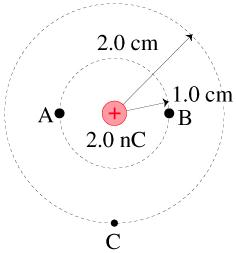# Problem: a. What is the electric potential at points A, B, and C in the figure?b. What is the potential energy of an electron at point A in the figure?c. What is the potential energy of an electron at point B in the figure?d. What is the potential energy of an electron at point C in the figure?e. What is the potential difference ΔVAB?f. What is the potential difference ΔVBC?

###### FREE Expert Solution

a.

Electric potential:

$\overline{){\mathbf{V}}{\mathbf{=}}\frac{\mathbf{k}\mathbf{q}}{\mathbf{r}}}$

At point A,

79% (19 ratings)###### Problem Detailsa. What is the electric potential at points A, B, and C in the figure?

b. What is the potential energy of an electron at point A in the figure?

c. What is the potential energy of an electron at point B in the figure?

d. What is the potential energy of an electron at point C in the figure?

e. What is the potential difference ΔVAB?

f. What is the potential difference ΔVBC?